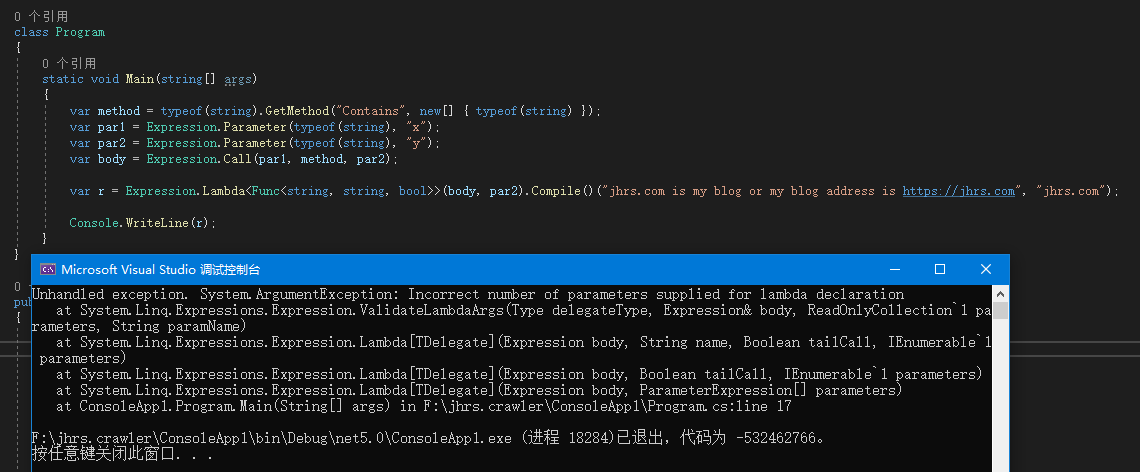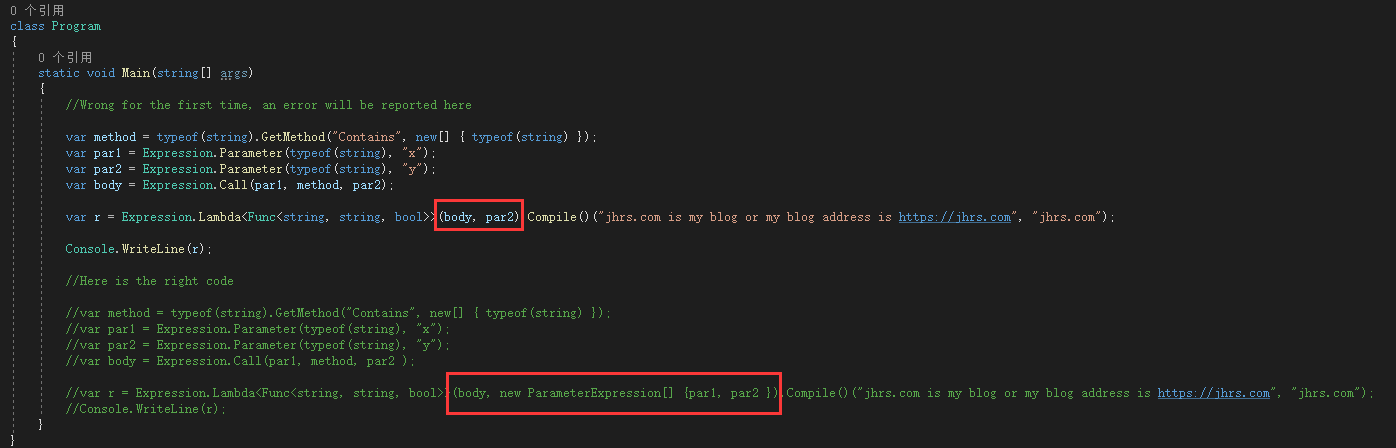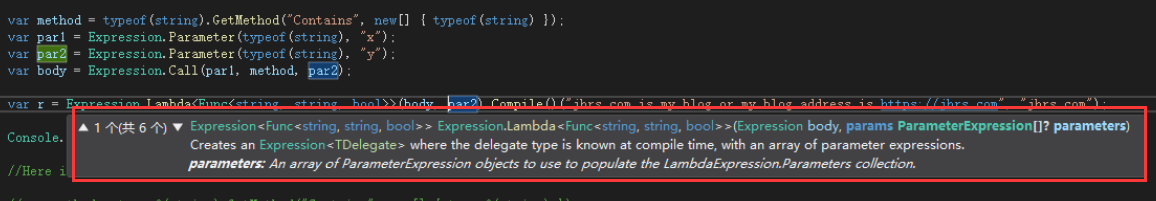# How to use Expression to call the Contains method of a string

How to use Expression to call the Contains method of a string? Recently, I encountered a requirement in a project that needs to dynamically use the expression Expression to call the Contains method of the string, and record a mistake made by the C# constructing Expression to call the string Contains.

In the initial code, because the parameters are written a bit, it is wasteful I spent some time searching for the cause, but I found it in the end, so make a note of the mistake I made.

If you are a C# developer, learning to use Expression will bring some benefits to writing some functions. For example, when we develop a paging function, we will have a lot of query conditions. For novices, these query conditions may be coded. It is handled by splicing SQL where conditions. When you learn to use expressions, this will be a very simple function.

The sample code in this post is derived from the code that I extracted when realizing server-side automatic legality verification of submitted form data based on asp.net , because one of the functions is to add custom attributes based on form controls Perform “>”, “<“, “=”, “>=”, “<=”, “!=” operations to verify whether the value of the control is legal. This is the best case for using Expression. Of course, if it is ASP In .NET Core, we can also use Expression to achieve very useful functions.How to use Expression to call the Contains method of a string

## Expression to call the Contains

Recently, I encountered a requirement in the project. It was necessary to dynamically use the expression Expression to call the Contains method of the string, and record a mistake made by C# constructing the Expression to call the string Contains. In the initial code, because the parameters were written a bit, it was a waste I spent some time searching for the cause, but I found it in the end, so make a note of the mistake I made.

Below is my original code.

```class Program
{
static void Main(string[] args)
{
var method = typeof(string).GetMethod("Contains", new[] { typeof(string) });
var par1 = Expression.Parameter(typeof(string), "x");
var par2 = Expression.Parameter(typeof(string), "y");
var body = Expression.Call(par1, method, par2);

var r = Expression.Lambda<Func<string, string, bool>>(body, par2).Compile()("jhrs.com is my blog or my blog address is https://jhrs.com", "jhrs.com");

Console.WriteLine(r);
}
}```

Run the program and you will get the following error.How to use Expression to call the Contains method of a string

The error content is:

```Unhandled exception. System.ArgumentException: Incorrect number of parameters supplied for lambda declaration
at System.Linq.Expressions.Expression.ValidateLambdaArgs(Type delegateType, Expression& body, ReadOnlyCollection`1 parameters, String paramName)
at System.Linq.Expressions.Expression.Lambda[TDelegate](Expression body, String name, Boolean tailCall, IEnumerable`1 parameters)
at System.Linq.Expressions.Expression.Lambda[TDelegate](Expression body, Boolean tailCall, IEnumerable`1 parameters)
at System.Linq.Expressions.Expression.Lambda[TDelegate](Expression body, ParameterExpression[] parameters)
at ConsoleApp1.Program.Main(String[] args) in F:\jhrs.crawler\ConsoleApp1\Program.cs:line 17```

## What is the cause of the error

When I saw the error shown in the figure above, I wasted some time looking for the cause of the error. The reason I finally located was the parameter transmission error. After I wrote the correct code, I could clearly find that it was the same as the original one. The difference in code.

The following shows you the correct way of writing:

```class Program
{
static void Main(string[] args)
{
var method = typeof(string).GetMethod("Contains", new[] { typeof(string) });
var par1 = Expression.Parameter(typeof(string), "x");
var par2 = Expression.Parameter(typeof(string), "y");
var body = Expression.Call(par1, method, par2);

var r = Expression.Lambda<Func<string, string, bool>>(body, new ParameterExpression[] { par1, par2 }).Compile()("jhrs.com is my blog or my blog address is https://jhrs.com", "jhrs.com");
Console.WriteLine(r);
}
}```

Well, let’s compare and see what is the difference:How to use Expression to call the Contains method of a string
```class Program
{
static void Main(string[] args)
{
//Wrong for the first time, an error will be reported here

var method = typeof(string).GetMethod("Contains", new[] { typeof(string) });
var par1 = Expression.Parameter(typeof(string), "x");
var par2 = Expression.Parameter(typeof(string), "y");
var body = Expression.Call(par1, method, par2);

var r = Expression.Lambda<Func<string, string, bool>>(body, par2).Compile()("jhrs.com is my blog or my blog address is https://jhrs.com", "jhrs.com");

Console.WriteLine(r);

//Here is the right code

//var method = typeof(string).GetMethod("Contains", new[] { typeof(string) });
//var par1 = Expression.Parameter(typeof(string), "x");
//var par2 = Expression.Parameter(typeof(string), "y");
//var body = Expression.Call(par1, method, par2 );

//var r = Expression.Lambda<Func<string, string, bool>>(body, new ParameterExpression[] {par1, par2 }).Compile()("jhrs.com is my blog or my blog address is https://jhrs.com", "jhrs.com");
//Console.WriteLine(r);
}
}```

How to use Expression to call the Contains method of a string

The reason for the error is as I used the red mark in the above picture, because the wrong parameter was given.

## in conclusion

After I solved this problem, I came back to review this problem and found that I made a low-level mistake. In fact, in the process of writing code, if you are writing this program in Visual Studio 2019 , you only need to use the shortcut keys. CTRL+SHIFT+SPACE can get the prompt as shown below.How to use Expression to call the Contains method of a string

Hope you have fun coding.